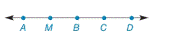Chapter 2.2, Problem 28EElementary Geometry For College St...

7th Edition
Alexander + 2 others
ISBN: 9781337614085

Solutions

Chapter
SectionElementary Geometry For College St...

7th Edition
Alexander + 2 others
ISBN: 9781337614085
Textbook Problem

In Exercises 25 to 36, give the indirect proof for each problem or statement. Given: M B > B C A M = C D Prove: B is not themidpoint of A D ¯To determine

To prove:

The given statement.

Explanation

Given:

The given statement is,

MB>BC and AM=CD

Figure (1)

Property:

A point B lies between the points A and C of line segment AC if and only if, AB+BC=AC.

Approach:

Assume that B is the midpoint of AD¯.

AB¯=BD¯

Point M lies between the points A and B. Point C lies between the points B and D in figure (1).

By above stated property,

AB¯=AM¯+MB¯BD¯=BC¯+CD¯AM¯+MB¯=BC¯+CD¯

Still sussing out bartleby?

Check out a sample textbook solution.

See a sample solution

The Solution to Your Study Problems

Bartleby provides explanations to thousands of textbook problems written by our experts, many with advanced degrees!

Get Started

Find more solutions based on key concepts